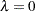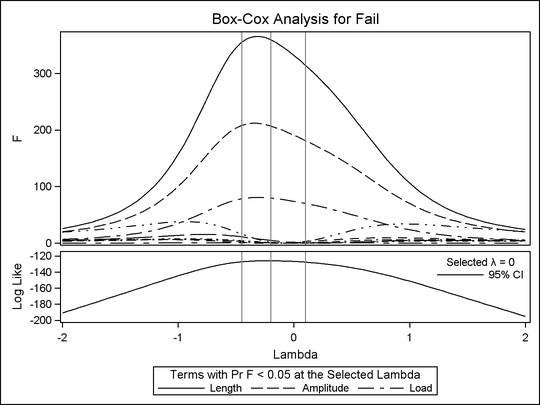Statistical Graphics Using ODS
 Box-Cox Transformation Plot with PROC TRANSREG

This example is taken from Example 91.2 of Chapter 91, The TRANSREG Procedure. The following statements create a SAS data set that contains failure times for yarn:

```proc format;
value a -1 =   8 0 =   9 1 =  10;
value l -1 = 250 0 = 300 1 = 350;
value o -1 =  40 0 =  45 1 =  50;
run;

data yarn;
input Fail Amplitude Length Load @@;
format amplitude a. length l. load o.;
label fail = 'Time in Cycles until Failure';
datalines;
674 -1 -1 -1    370 -1 -1  0    292 -1 -1  1    338  0 -1 -1

... more lines ...

;
```

The following statements set the output style to JOURNAL2 and run PROC TRANSREG:

```ods listing style=journal2;
ods graphics on;

proc transreg data=yarn;
model BoxCox(fail / convenient lambda=-2 to 2 by 0.05) =
The log-likelihood plot in Figure 21.9 suggests a Box-Cox transformation with.